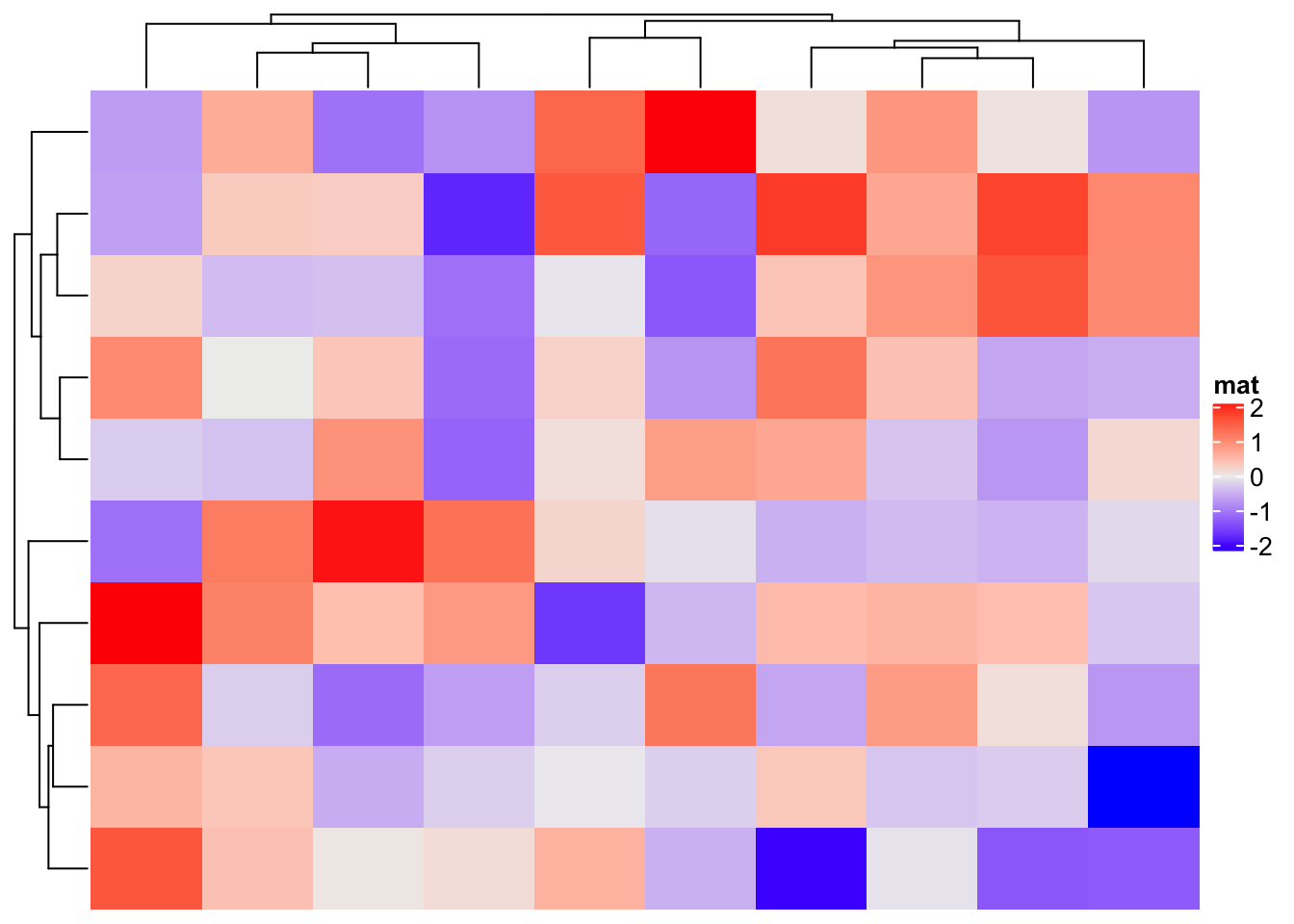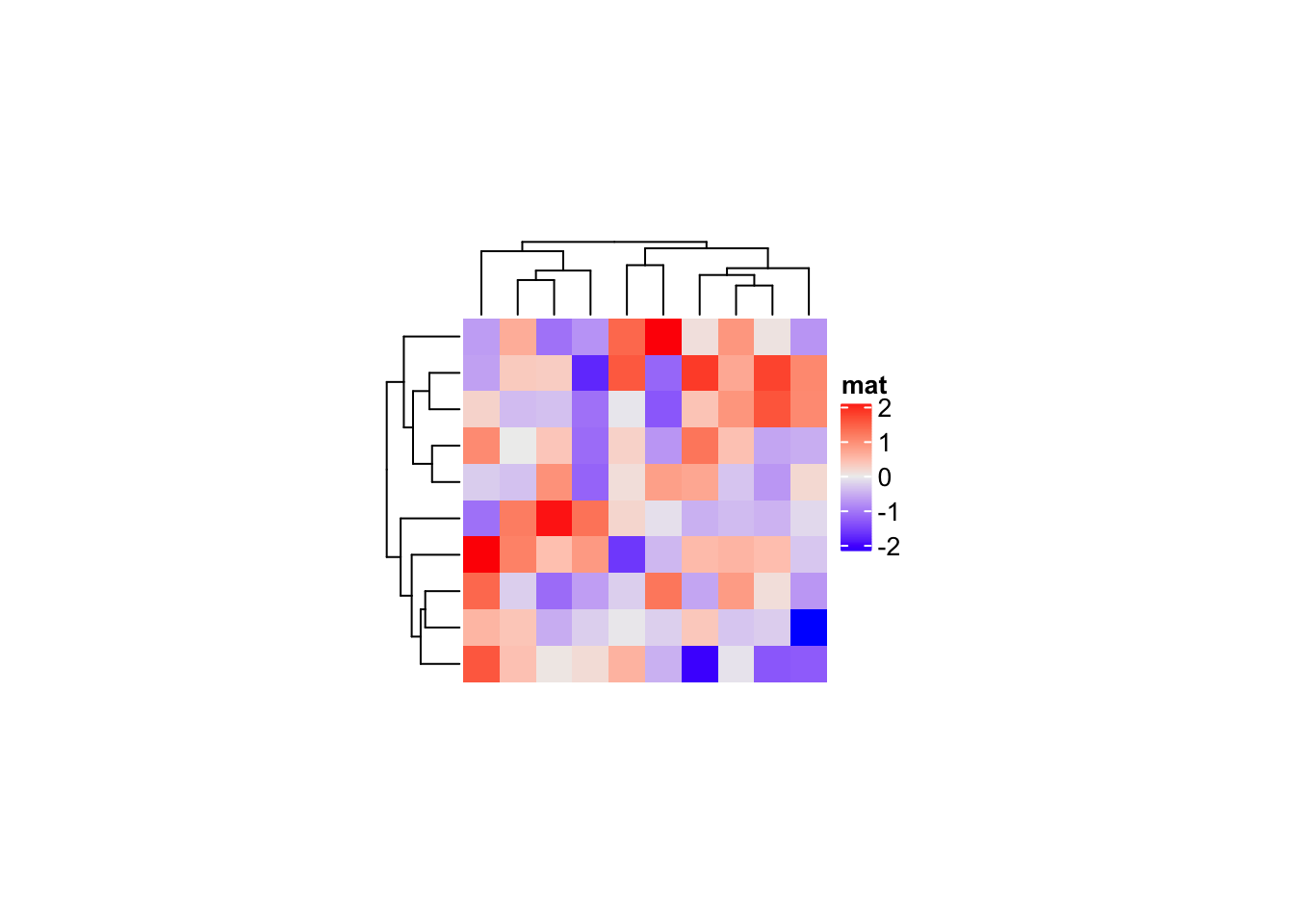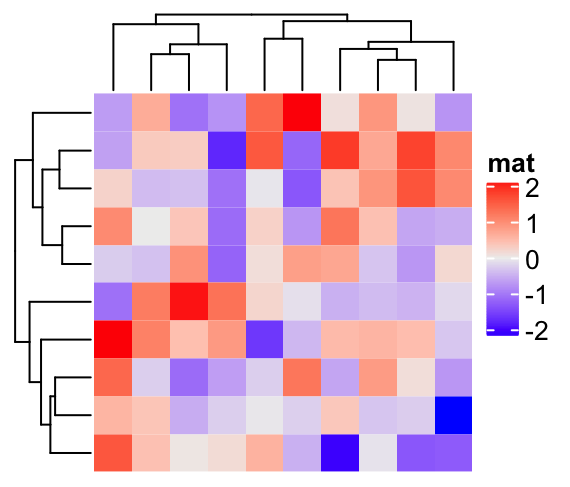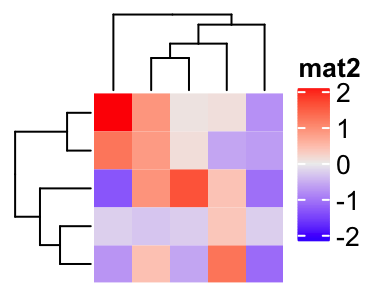# Set cell width/height in the heatmap

When making a heatmap, the width and height of heatmap cells are automatically adjusted to fill in the figure (e.g. the following figure), which means, when you change the width and height of the final figure, the width and height of the cells change accordingly.

``````library(ComplexHeatmap)
set.seed(123)
mat = matrix(rnorm(100), 10)
Heatmap(mat, name = "mat")``````When the matrix is small, there are scenarios where you might want to manually set the width and height of cells to fixed values, so that you can, e.g., put proper row/column labels or to make it easy to compare to other heatmaps with different dimensions.

In `Heatmap()` function, the total width and height of the heatmap body can be controlled by the `width` and `height` arguments, which means you can control the size of the whole heatmap by setting proper values to these two arguments. E.g. setting `width` to `ncol(mat)*unit(5, "mm")` and `height` to `nrow(mat)*unit(5, "mm")` ensures the cells to be 5mm for both width and height.

To save the plot into an figure file, one problem arising is you need to properly calculate the size of the figure to completely place the plot, or else the plot is either clipped or there has white spaces around the plot.

In the following figures, the size of the figure is larger than the size of the heatmap. A black border is added to show the size of the figure.

``````Heatmap(mat, name = "mat",
width = ncol(mat)*unit(5, "mm"),
height = nrow(mat)*unit(5, "mm"))``````In ComplexHeatmap, the size of the heatmap (including dendrograms, titles and legends, …) can be retrieved by `ComplexHeatmap:::width()` and `ComplexHeatmap:::height()` functions. Note these two functions currently are not exported, so you need to use `:::` to use them.

To use `ComplexHeatmap:::width()` and `ComplexHeatmap:::height()`, you first need to explicitly execute `draw()` function to perform clustering and initialize the layout, then `ComplexHeatmap:::width()` and `ComplexHeatmap:::height()` and be applied and they return the corresponding width and height as `grid::unit` objects in mm. You can convert to any other units by `grid::convertX()` and `grid::convertY()` functions.

``````ht = Heatmap(mat, , name = "mat",
width = ncol(mat)*unit(5, "mm"),
height = nrow(mat)*unit(5, "mm"))
ht = draw(ht) # it generates a plot, but we don't show here``````
``````w = ComplexHeatmap:::width(ht)
w = convertX(w, "inch", valueOnly = TRUE)
h = ComplexHeatmap:::height(ht)
h = convertY(h, "inch", valueOnly = TRUE)
c(w, h)``````
``##  2.973294 2.539370``

Now `w` and `h` are the sizes of the heatmap, measured in inches which you can set in `pdf()` function. If you save the plot as a PNG plot, then you need to convert `w` and `h` to a unit of `pt` (see the help page of `grid::unit`).

``````# pdf(..., width = w, height = h)
Heatmap(mat, name = "mat",
width = ncol(mat)*unit(5, "mm"),
height = nrow(mat)*unit(5, "mm"))````````# dev.off()``

The calculation of heatmap size actually can be wrapped as a function, which takes a `Heatmap` or `HeatmapList` object as input and returns the corresponding width and height.

In the following `calc_ht_size()` function, since we don’t need the first heatmap, we put it into a null device defined by `pdf(NULL)`.

``````calc_ht_size = function(ht, unit = "inch") {
pdf(NULL)
ht = draw(ht)
w = ComplexHeatmap:::width(ht)
w = convertX(w, unit, valueOnly = TRUE)
h = ComplexHeatmap:::height(ht)
h = convertY(h, unit, valueOnly = TRUE)
dev.off()

c(w, h)
}``````

Now we can try to use `calc_ht_size()`

``````ht = Heatmap(mat, name = "mat",
width = ncol(mat)*unit(5, "mm"),
height = nrow(mat)*unit(5, "mm"))
size = calc_ht_size(ht)
size``````
``##  2.973294 2.539370``
``````# pdf(..., width = size, height = size)
ht````````# dev.off()``

Similar for a smaller matrix:

``````mat2 = mat[1:5, 1:5]
ht2 = Heatmap(mat2, name = "mat2",
width = ncol(mat2)*unit(5, "mm"),
height = nrow(mat2)*unit(5, "mm"))
size2 = calc_ht_size(ht2)
size2``````
``##  1.989042 1.555118``
``````# pdf(..., width = size2, height = size2)
ht2 # or draw(ht2)````````# dev.off()``